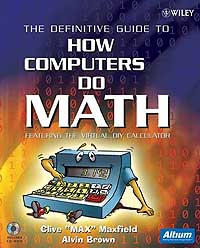# Rounding

Also found in: Dictionary, Thesaurus, Medical, Idioms, Wikipedia.

## rounding

[′rau̇nd·iŋ]
(mathematics)
Dropping or neglecting decimals after some significant place. Also known as truncation.

## Rounding

The rounding of a number is the approximate representation of the number in some numerical notation by means of a finite number of digits. Rounding is necessitated by the requirements of computation, in which a final result generally cannot be obtained with absolute accuracy and the purposeless writing out of superfluous digits should be avoided by limiting all numbers to just the necessary quantity of characters. When a number is rounded, it is transformed into another number with t digits that is an approximate representation of it. The resulting error is called the rounding, or round-off, error.

Various methods of rounding are used. The simplest, truncation, consists of discarding the low-order digits of a number after the t th digit. The absolute error in this case does not exceed a unity in the t th place of the number. The rounding method usually used in hand calculations consists in rounding the number to the nearest t-place number. The absolute rounding error in this case does not exceed half of unity in the t th digit of the number. This method gives the minimum possible error of all methods that round to t places.

The rounding methods used in a computer are determined by the computer’s purpose and capabilities and as a rule are less precise than rounding to the nearest t-place number. The modes of arithmetic most widely used in digital computers are floating point and fixed point. In floating point arithmetic the rounded result has a fixed number of significant digits, whereas in fixed point arithmetic it has a fixed number of digits after the decimal point. In the first case we say we are rounding to t places, and in the second case, to t places after the decimal point. Also, in the first case the relative rounding error is controlled, whereas in the second case it is the absolute error that is controlled.

In connection with the use of computers, studies have been made of the accumulation of rounding errors in large-scale computations. The analysis of error accumulation in numerical methods permits characterization of the methods according to their sensitivity to rounding errors. It makes possible the creation of strategies for implementing the methods in computational practice—strategies that take rounding errors into account. Finally, such analysis makes possible estimation of the accuracy of final results.

### REFERENCES

Krylov, A. N. Lektsii o priblizhennykh vychisleniiakh, 6th ed. Moscow, 1954.
Berezin, I. S., and N. P. Zhidkov. Metody vychislenii, 3rd ed., vol. 1.
Moscow, 1966. Bakhvalov, N. S. Chislennye metody. Moscow, 1973.

G. D. KIM

## round

To eliminate rightmost digits in a number when absolute precision is not required or used. One of the most common uses of rounding is with dollar amounts, which can result in more than two decimal places after a division. Following are four of many rounding methods:

```  Round Half Up    3.455 -> 3.46
3.454 -> 3.45

Round Half Down  3.455 -> 3.45
3.456 -> 3.46

Round Up         3.456 -> 3.46
3.453 -> 3.46

Round Down       3.458 -> 3.45
3.453 -> 3.45
```

Rounding and a Lot MoreFor more ways to round numbers than you can imagine, as well as to learn how computers perform mathematical functions at the circuit level, read the entertaining and informative book, "How Computers Do Math" by Clive "Max" Maxfield and Alvin Brown. (John Wiley & Sons, Inc. 2005).
Site: Follow: Share:
Open / Close